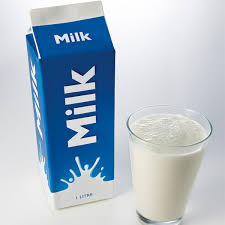# Milky & Tricky

Geometry Level 2You have bought 1200 cm$^3$ of milk. You poured all of the milk into a container whose base is a rectangle measuring 12cm $\times$ 10cm.

Then you decided that you would heat up some milk and you poured some of it into a pot that has a bottom area of 80 centimeter squared. Then you discovered that the height of milk in both containers are the same. How much milk, in cm$^3$, is in the pot?

×